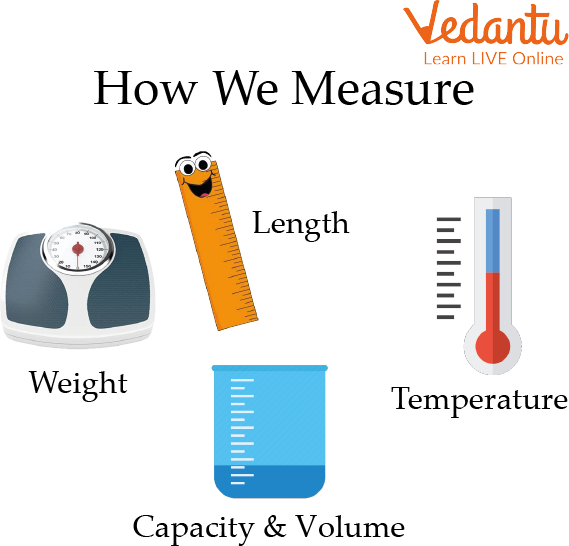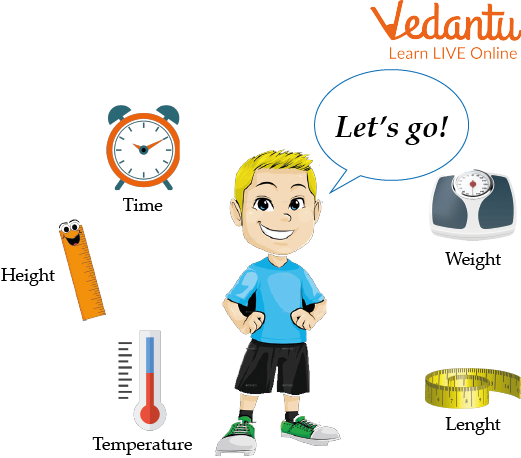Courses
Courses for Kids
Free study material
Free LIVE classes
More

# Temperature, Capacity, Weight and MassLIVE
Join Vedantu’s FREE Mastercalss

## A Brief Explanation of Temperature, Capacity, Weight and Mass

This article mainly presents a brief description of Temperature, Capacity, Weight and Mass, which we see in our daily life. It is also used in some way or the other in our daily work, children need to know about it. Some of these things have been taken care of in this article, we are going to discuss some definitions related to it and the mass and weight difference, along with vital capacity and units to measure capacity. Also, examples and worksheets related to them have been given, which makes it easier to understand. Taking this sequence forward, we try to understand this article.Units to Measure Capacity

## Difference Between Mass and Weight

Here the mass and weight differences are explained:

 Weight Mass If there is no gravitational pull on an item, as in space, weight can be zero. There can never be zero mass. A vector quantity is a weight. It is aimed at the centre of the Earth or another gravity well and has magnitude. A scalar quantity is a mass. It is substantial. The force unit of newtons is frequently used to measure weight. In most cases, mass is expressed in grams and kilogrammes. The impact of gravity affects weight. With a higher or lower gravity, weight rises or falls. A characteristic of matter is mass. An object's mass is constant everywhere. Weight varies geographically. Regardless of location, mass remains constant. A spring balance is used to calculate the weight. Use a standard balance to calculate mass.Different Units of Measurement

## Vital Capacity

When anything is referred to as vital, it denotes that it is necessary or extremely significant. The total amount of air that can be expended from the lungs with the greatest amount of exertion is known as the vital capacity(VC). It enables simultaneous inhalation of the greatest possible volume of clean air and exhalation of the greatest possible volume of stale air. So, by increasing gaseous exchange between the body's various tissues, it improves the amount of energy available for bodily function.

## Units to Measure Capacity

Any container's capacity refers to the volume of liquid it can hold. The United States Customary Units is another name for the American metric system (USCS). USGS measures the capacity using 5 standardized units. A liquid's volume is expressed using the phrase capacity. Other units that are multiples and submultiples of litres are also used to measure big and tiny quantities. The larger and more compact units that are connected to litres are shown in the following table:

 Litres Different units of conversion 0.001 litre (l) Millilitre (ml) 0.01liter (l) Centiliter (cl) 0.1 litre (l) Deciliter (dl) 10 litres (l) Decaliter (dal) 100 litres (l) Hectoliter (hl) 1000 litres (l) Kiloliter (kl)

 Units of Capacity Example Cup Tea or Coffee Quart Water Jar or Milk Carton Fluid Ounce Syrup or Tonic Gallon Gasoline or Petrol Pint Juice Jar

## Temperature, Capacity, Weight and Mass Related Solved Examples

Here are some questions mainly related to the conversion of units, which are like this;

Example 1. Convert 300 kilometres in meters.

Solution: We know that 1 km = 1000 m

So, 1 km ×100= 100 kilometres

Putting the value of 1km = 1000 m

100 km = 1000 m × 100

= 100000m

So, 300 km = 300000 m.

Therefore, 300 km equals 300000 m.

Example 2. Convert 45 degree Celsius to Fahrenheit?

Solution- Given us, Temperature = 45 degree Celsius

We know that,

F = 9/5 X C + 32

F = 9/5 X 45 + 32

F= 9 X 5 + 32

F= 45 + 32

F= 77 ° F

Therefore, 45-degree celsius equals 77 degrees Fahrenheit.

Example 3. 7 kilograms is equal to how many grams?

Solution- Given us, 7 kilograms is changed into grams.

We know that,

1 kilogram = 1000 gram

So,

7 kilograms = 7 X 1000 gram

7 kilograms = 7000 gram

Therefore, 7 kg equals 7000 g.

## Practice Problems

Here are some practice problems to be solved by the students themselves:

1. Convert 65 degree Celsius to Fahrenheit?

Ans. 149 ° F.

2. 41 kilograms is equal to how many grams?

Ans. 41000 gms.

3. Convert 3 kilometres in meters.

Ans. 3000 m.

4. Convert 77⁰ Fahrenheit to Celsius?

Ans. 25 degrees Celsius.

## Summary

Capacity, Weight/Mass, and Temperature are the three main factors that affect one's life. It enables kids to precisely and properly estimates the object's dimensions. Additionally, it makes determining an object's size interesting and engaging for learning about the issue at hand. Here, children have learned about the difference between mass and weight along with vital capacity and units to measure capacity. This article has been made keeping these few things in mind, where not only examples related to the conversion of units have been given but practice problems related to them have also been presented, which play a major role in the development of children.

Last updated date: 01st Oct 2023
Total views: 99.6k
Views today: 1.99k

## FAQs on Temperature, Capacity, Weight and Mass

1. What are the three standard units of measurement?

The inch, foot, yard, and mile are the fundamental units of length and distance measurement in the English system.

2. What different capacities are there?

Design capacity, effective capacity, and actual capacity are the three criteria used to define capacity. These can then be used to calculate how effectively the activities use their resources and how efficiently their processes work.

3. Is capacity the same as volume?

The total amount of space an object occupies in three dimensions is indicated by its volume. The term "capacity" describes something's ability to contain, absorb, or receive by an object (such as a solid substance, gas, or liquid).